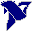# LabVIEW

cancel
Showing results for
Search instead for
Did you mean:

## Curve fit for sensor data

1. Have a thermistor, data whose dataset is available not anything else from company except B value. Attached is data.   Resistance is "x" & temperature is "y"

Need to fit y =F(x)

2. Trying to make a equation for it and implement for 8 bit MCU, usin IAR IDE.

3. First I made this code, steinhart thermistor equation. calculated values of A,B,C from this site:  https://www.thinksrs.com/downloa...  . Put three values at -20C, 25C & 100C and get A,B,C

Its involves natural log and can fit data points, but I have to implement it on 8 bit MCU so it takes lots of space on it.

4. Then I used labview tool, to make equation , I have to break it down dataset into multiple sets for fitting 2nd order equation.

Here are coefficients for resistance values I got, for 2nd order

```    if( (resistance <= 956040.0f) &&  (resistance >= 482170.0f) )
{
c0 = 14.5029518320319131f;
c1 = -0.000057344817030149406f;
c2 = 0.000000000021788855747503695f;
}
else if(resistance >= 250140.0f)
{
c0 = 29.3136847463533279f;
c1 = -0.000119496884518122221f;
c2 = 0.0000000000876747221220605721f;
}
else if(resistance >= 130230.0f)
{
c0 = 45.3985586636038647f;
c1 = -0.000249401966454676711f;
c2 = 0.000000000352768556658954028f;
}
else if(resistance >= 71230.0f)
{
c0 = 62.1448935476119572f;
c1 = -0.000506560472110208432f;
c2 = 0.00000000135019658772898851f;
}
else if(resistance >= 39200.0f)
{
c0 = 79.7753263268828902f;
c1 = -0.00100556530593662709f;
c2 = 0.00000000491385322365418885f;
}
else if(resistance >= 21830.0f)
{
c0 = 98.8979251125107339f;
c1 = -0.00198872984475097995f;
c2 = 0.0000000176669521860767602f;
}
else if(resistance >= 12750.0f)
{
c0 = 118.809289117525665f;
c1 = -0.00381338043851388829f;
c2 = 0.0000000598289053823529209f;
}
else if(resistance >= 7327.0f)
{
c0 = 140.313484336487619f;
c1 = -0.00722784306211046376f;
c2 = 0.000000196440993753396293f;
}
else if(resistance >= 6683.0f)
{
c0 = 133.215333415477943f;
c1 = -0.00480709971651089099f;
c2 = 0.0f;
} ```

Any other method, where I get better fit equation or lesser sub datasets I have to break to?

Message 1 of 6
(638 Views)

## Re: Curve fit for sensor data

Hi Vindhyachal,

with sensors like thermistors (NTC or PTC type) you often use additional resistors to form voltage dividers with the thermistor and also to linearize the voltage=f(temperature) behaviour, as described in this AppNote.

This Appnote also shows methods to convert the voltage signal back to a temperature:

• apply a polynomial on the linearized voltage signal
• using a LUT in the linearized voltage signal

For the NTCs I work with I choose the linearization resistor based on (mainly) two considerations:

• current through NTC to prevent self-heating
• best linearization behaviour depending on the expected typical temperature range to be measured
Best regards,
GerdWusing LV2011SP1 + LV2017 (+LV2020 sometimes) on Win10+cRIO
Message 2 of 6
(625 Views)

## Re: Curve fit for sensor data

Here is VI I made

It keep on decreasing dataset values unless error is less than defined error. This way i can divide equation into multiple parts.

Message 3 of 6
(619 Views)

## Re: Curve fit for sensor data

Hi Vindhyachal,

use the data to create a LUT, then interpolate linearly between two neighboring data points (should be accurate enough for a NTC sensor):To improve code performance you need to create the LUT only once: all the code until Reverse1DArray should only be called once. You can even create a constant of the data after Reverse1DArray…

Best regards,
GerdWusing LV2011SP1 + LV2017 (+LV2020 sometimes) on Win10+cRIO
Message 4 of 6
(612 Views)

## Re: Curve fit for sensor data

@gerdw can u share the VI.

Dont have much exp in coding in labview. Could nt find the image icons some of them

Message 5 of 6
(557 Views)

## Re: Curve fit for sensor data

Hi Vindhyachal,

@Vindhyachal.Takniki wrote:

can u share the VI.

Dont have much exp in coding in labview. Could nt find the image icons some of them

It's a snippet. Read the LabVIEW help on "snippets"…

There are only 5 simple functions:

• SpreadsheetStringToArray
• IndexArray
• Index&BundleClusterArray
• Reverse1DArray
• Interpolate1DArray
Best regards,
GerdWusing LV2011SP1 + LV2017 (+LV2020 sometimes) on Win10+cRIO
Message 6 of 6
(554 Views)